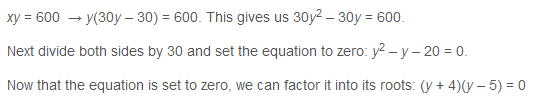## Articles tagged "algebra"

### What’s Tested on GRE MathGRE Math is a bit like high school math, without some of the hardest parts: for instance, you don’t have to write proofs or show your work! Here’s a quick rundown of the GRE Math skills required to conquer the Quant section, along with some of our best GRE Math tips. Read more

### Manhattan GRE’s Halloween Challenge Problem

We hope everyone had a happy Halloween! Yesterday we asked our friends on our Manhattan GRE Facebook page to attempt this Trick-or-Treat Halloween Challenge Problem. As promised, today we are sharing the answer and explanation to the problem:Let’s use x for the number of bags produced by the original recipe, and for the weight of each of the bags. Given those variables, our first equation is simply xy = 600. We also need to create an equation that represents the new recipe. Since the number of bags produced has increased by 30, and the weight of each bag has decreased by 1, the new equation is (x + 30)(y – 1) = 600. Remember, the total weight is still 600 ounces. Foiling this equation yields xy – x + 30y – 30 = 600.

We now have two equations with two variables. There are several different paths we can go down here, but all involve substitution of one of the variables, and all will yield a quadratic. The simplest path is to recognize that since xy = 600, we can substitute for xy in the second equation to get 600 – x + 30y – 30 = 600. Subtracting the 600 from both sides, and adding an x to each side gives us 30y – 30 = x. We can now substitute for x in the first equation.### Using Smart Numbers to Avoid Algebra on the GRE

Many word problems seem to require us to write formulas in order to solve. Certain problems, though, qualify for a neat technique: Smart Numbers. We can actually pick our own real numbers and use them to solve!

Set your timer for 2 minutes for this Fill-In problem and GO! (© ManhattanPrep)

* Lisa spends 3/8 of her monthly paycheck on rent and 5/12 on food. Her roommate, Carrie, who earns twice as much as Lisa, spends ¼ of her monthly paycheck on rent and ½ on food. If the two women decide to donate the remainder of their money to charity each month, what fraction of their combined monthly income will they donate? (Assume all income in question is after taxes.)

(No answer choices given; this is a fill-in-the-blank)We’ve got two women, Lisa and Carrie, and they each spend a certain proportion of income on rent and on food. Annoyingly, the fractions don’t have the same denominators; even more annoyingly, the two women don’t make the same amount of money. All of that will make an algebraic solution challenging.

Here’s what an algebraic solution would look like. Let’s call Lisa’s income x. She spends (3/8)x on rent and (5/12)x on food. Add these together:

(3x/8) + (5x/12) = (9x/24) + (10x/24) = 19x/24

Subtract from 100%, or x:

24x/24 “ 19x/24 = 5x/24

Lisa donates 5/24 of x, her income, to charity. What about Carrie?

Carrie’s income is equal to 2x (because she makes twice as much as Lisa). How much does she spend on rent and food?